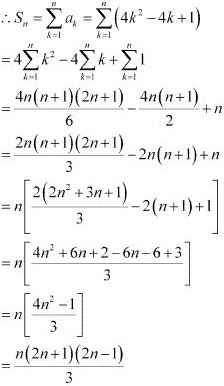# Class 11 Maths NCERT Solutions for Chapter 9 Sequences and Series Exercise 9.4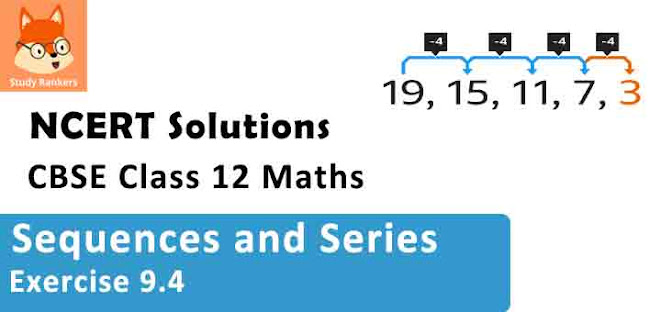### Sequences and Series Exercise 9.4 Solutions

1. Find the sum to n terms of the series 1 × 2 + 2 × 3 + 3 × 4 + 4 × 5 + …

Solution

The given series is 1 × 2 + 2 × 3 + 3 × 4 + 4 × 5 + …
nth term, an = n ( n + 1)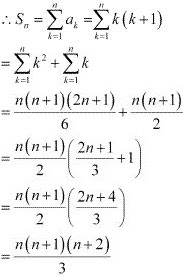2. Find the sum to n terms of the series 1 × 2 × 3 + 2 × 3 × 4 + 3 × 4 × 5 + …

Solution

The given series is 1 × 2 × 3 + 2 × 3 × 4 + 3 × 4 × 5 + …
nth term, an = n ( n + 1) ( n + 2)
= (n2 + n) (n + 2)
= n+ 3n+ 2n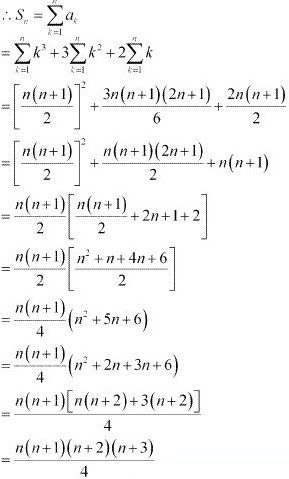3. Find the sum to n terms of the series 3 × 12 + 5 × 22 + 7 × 32 + …

Solution

The given series is 3 ×12 + 5 × 22 + 7 × 32 + …
nth term, an = ( 2n + 1) n2 = 2n3 + n2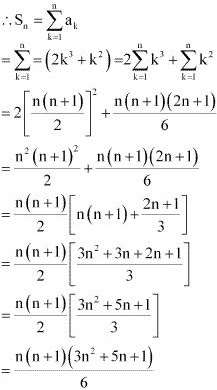4. Find the sum to n terms of the series 1/(1× 2) + 1/(2× 3) + 1/(3× 4) + .....

Solution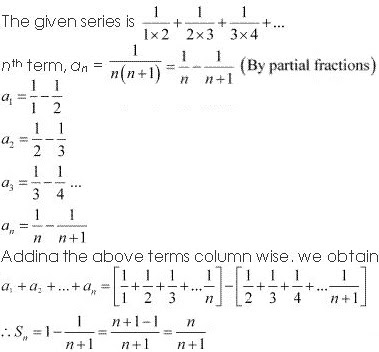5. Find the sum to n terms of the series 52 + 62 + 72 + ... + 202 .
Solution
The given series s 52 + 62 + 72 + .... + 202
nth term, an = ( n + 4)2 = n2 + 8n + 16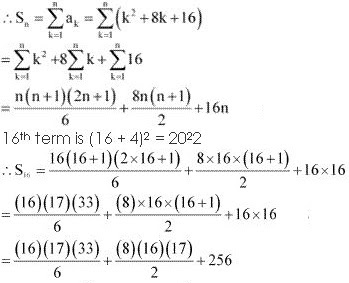= 1496 + 1088 + 256
= 2840
∴ 52 + 62 + 72 + ... + 202 = 2840

6. Find the sum to n terms of the series 3 × 8 + 6 × 11 + 9 × 14 +…
Solution
The given series is 3 × 8 + 6 × 11 + 9 × 14 + …
a= (nth term of 3, 6, 9 …) × (nth term of 8, 11, 14, …)
= (3n) (3n + 5)
= 9n2 + 15n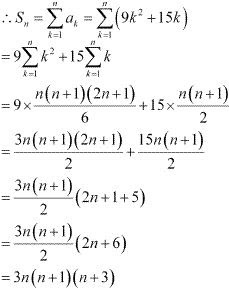7. Find the sum to n terms of the series 12 + (12 + 22) + (12 + 22 + 32) + …

Solution

The given series is 12 + (12 + 22) + (12 + 2+ 32 ) + …
an = (12 + 22 + 32 +….+ n2)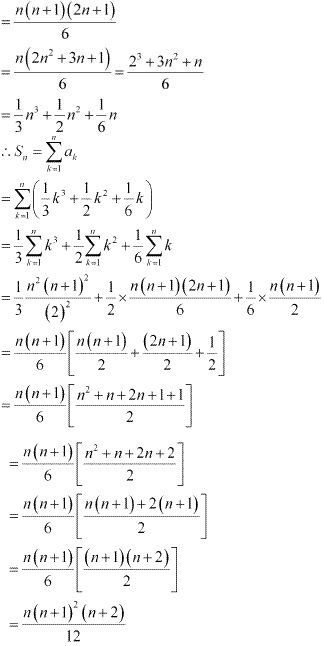8. Find the sum to n terms of the series whose nth term is given by n (n + 1) (n + 4).

Solution

9. Find the sum to n terms of the series whose nth terms is given by n2 + 2n.

Solution

an = n2 + 2n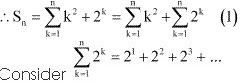The above series 2, 22, 23, .... is a G.P. with both the first term and common ratio equal to 2.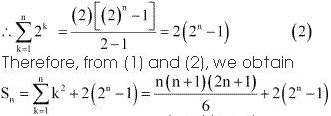10. Find the sum to n terms of the series whose nth terms is given by (2n – 1)2 .

Solution

an (2n – 1)2 = 4n2 – 4n + 1